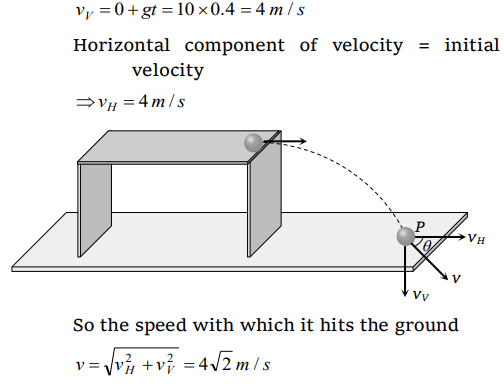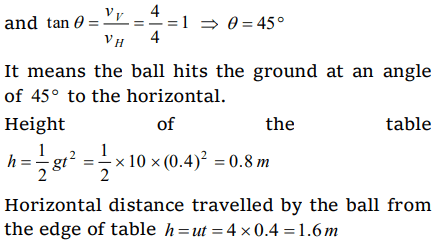## Motion in a Plane Questions and Answers Part-17

1. The maximum range of a gun on horizontal terrain is 16 km. If $g=10m\diagup s^{2}$  . What must be the muzzle velocity of the shell
a) 200 m/s
b) 400 m/s
c) 100 m/s
d) 50 m/s

Explanation:2. A stone is just released from the window of a train moving along a horizontal straight track. The stone will hit the ground following
a) Straight path
b) Circular path
c) Parabolic path
d) Hyperbolic path

Explanation: Due to constant velocity along horizontal and vertical downward force of gravity stone will hit the ground following parabolic path

3. A bullet is dropped from the same height when another bullet is fired horizontally. They will hit the ground
a) One after the other
b) Simultaneously
c) Depends on the observer
d) None of the above

Explanation: Because the vertical components of velocities of both the bullets are same and equal to zero and t = $\sqrt{\frac{2h}{g}}$

4. An aeroplane is flying at a constant horizontal velocity of 600 km/hr at an elevation of 6 km towards a point directly above the target on the earth's surface. At an appropriate time, the pilot releases a ball so that it strikes the target at the earth. The ball will appear to be falling
a) On a parabolic path as seen by pilot in the plane
b) Vertically along a straight path as seen by an observer on the ground near the target
c) On a parabolic path as seen by an observer on the ground near the target
d) On a zig-zag path as seen by pilot in the plane

Explanation: The pilot will see the ball falling in straight line because the reference frame is moving with the same horizontal velocity but the observer at rest will see the ball falling in parabolic path.

5. A bomb is dropped from an aeroplane moving horizontally at constant speed. When air resistance is taken into consideration, the bomb
a) Falls to earth exactly below the aeroplane
b) Fall to earth behind the aeroplane
c) Falls to earth ahead of the aeroplane
d) Flies with the aeroplane

Explanation: Due to air resistance, it’s horizontal velocity will decrease so it will fall behind the aeroplane.

6. A man projects a coin upwards from the gate of a uniformly moving train. The path of coin for the man will be
a) Parabolic
b) Inclined straight line
c) Vertical straight line
d) Horizontal straight line

Explanation: Because horizontal velocity is same for coin and the observer. So relative horizontal displacement will be zero

7. An aeroplane is flying horizontally with a velocity of 600 km/h at a height of 1960 m. When it is vertically at a point A on the ground, a bomb is released from it. The bomb strikes the ground at point B. The distance AB is
a) 1200 m
b) 0.33 km
c) 3.33 km
d) 33 km

Explanation:8. A ball is rolled off the edge of a horizontal table at a speed of 4 m/second. It hits the ground after 0.4 second. Which statement given below is true
a) It hits the ground at a horizontal distance 1.6 m from the edge of the table
b) The speed with which it hits the ground is 4.0 m/second
c) Height of the table is 0.8 m
d) Both a and c

Explanation: Vertical component of velocity of ball at point P9. An aeroplane flying 490 m above ground level at 100 m/s, releases a block. How far on ground will it strike
a) 0.1 km
b) 1 km
c) 2 km
d) None10. A body is thrown horizontally from the top of a tower of height 5 m. It touches the ground at a distance of 10 m from the foot of the tower. The initial velocity of the body is$\left(g=10 m s^{-2}\right)$
a) $2.5m s^{-1}$
b) $5m s^{-1}$
c) $10m s^{-1}$
d) $20m s^{-1}$